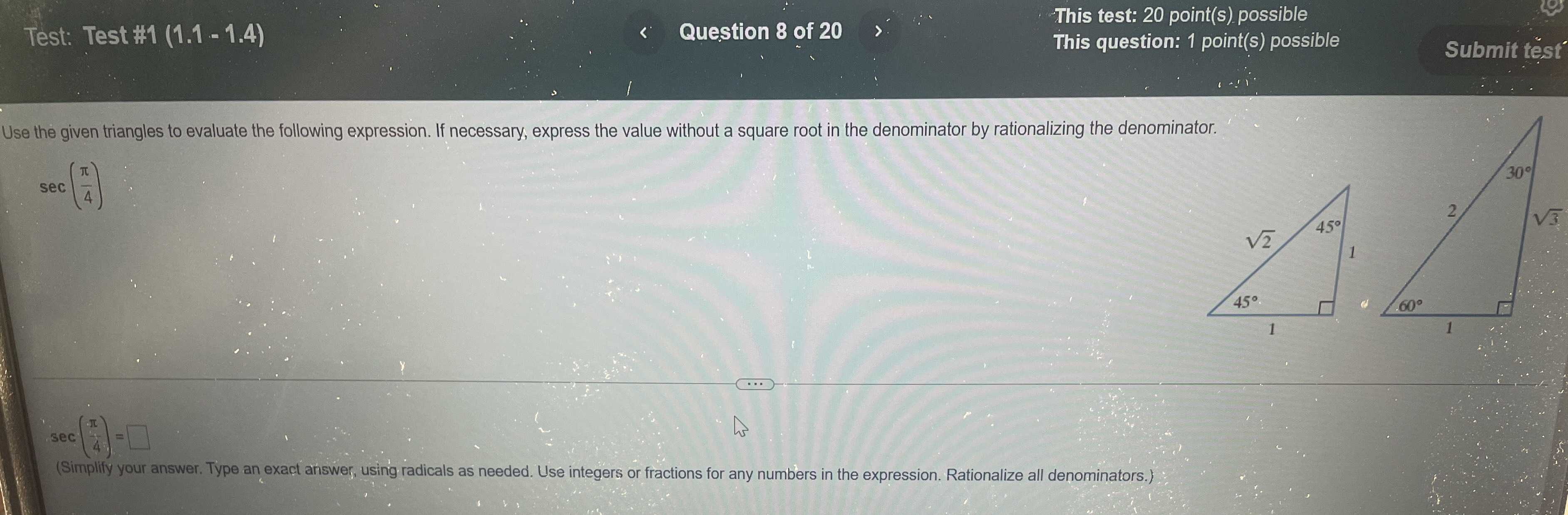### Still have math questions?

Trigonometry
QuestionUse the given triangles to evaluate the following expression. If necessary, express the value without a square root in the denominator by rationalizing the denominator.

$$\sec ( \frac { \pi } { 4 } )$$

$$\sec ( \frac { \pi } { 4} ) = \sqrt { 2}$$